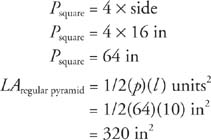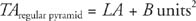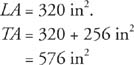## Regular Pyramids

regular pyramid is a pyramid whose base is a regular polygon and whose lateral edges are all equal in length. A pyramid is named by its base. Figure shows some examples of regular pyramids.Figure 1 Some different types of regular pyramids.

The lateral faces of a regular pyramid are congruent isosceles triangles. The altitude of any of these triangles is the slant height of the regular pyramid. Figure 2 is a square pyramid.Figure 2 A square pyramid.

Pyramids also have a lateral area, total area, and volume.

Theorem 93: The lateral area, LA, of a regular pyramid with slant height l and base perimeter p is given by the following equation.Example 1: Find the lateral area of the square pyramid, shown in Figure 3.Figure 3 Finding the lateral area, total area, and volume of a square pyramid.Because a pyramid has only one base, its total area is the sum of the lateral area and the area of its base.

Theorem 94: The total area, TA, of a regular pyramid with lateral area LA and base area B is given by the following equation.Example 2: Find the total area of the regular pyramid shown in Figure .

The base of the regular pyramid is a squareAsquare = (side)2. Therefore, B = 162 in2, or B = 256 in2.From the previous example,Theorem 95: The volume, V, of a regular pyramid with base area B and altitude h is given by the following equation.Example 3: Find the volume of the regular pyramid shown in Figure .

From the previous example, B = 256 in2. The figure indicates that h = 6 in.Back to Top
A18ACD436D5A3997E3DA2573E3FD792A